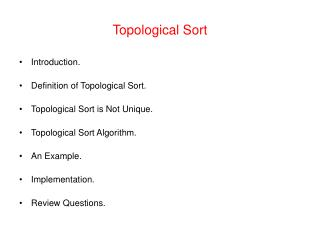DownloadDownload PresentationTopological Sort

# Topological Sort

Download Presentation## Topological Sort

- - - - - - - - - - - - - - - - - - - - - - - - - - - E N D - - - - - - - - - - - - - - - - - - - - - - - - - - -
##### Presentation Transcript

1. Topological Sort • Introduction. • Definition of Topological Sort. • Topological Sort is Not Unique. • Topological Sort Algorithm. • An Example. • Implementation. • Review Questions.

2. Introduction • There are many problems involving a set of tasks in which some of the tasks must be done before others. • For example, consider the problem of taking a course only after taking its prerequisites. • Is there any systematic way of linearly arranging the courses in the order that they should be taken? Yes! - Topological sort.

3. Definition of Topological Sort • Topological sort is a method of arranging the vertices in a directed acyclic graph (DAG), as a sequence, such that no vertex appear in the sequence before its predecessor. • The graph in (a) can be topologically sorted as in (b) (a) (b)

4. s1 = {a, b, c, d, e, f, g, h, i} s2 = {a, c, b, f, e, d, h, g, i} s3 = {a, b, d, c, e, g, f, h, i} s4 = {a, c, f, b, e, h, d, g, i} etc. Topological Sort is not unique • Topological sort is not unique. • The following are all topological sort of the graph below:

5. Topological Sort Algorithm • One way to find a topological sort is to consider in-degrees of the vertices. • The first vertex must have in-degree zero -- every DAG must have at least one vertex with in-degree zero. • The Topological sort algorithm is: • int topologicalOrderTraversal( ){ • int numVisitedVertices = 0; • while(there are more vertices to be visited){ • if(there is no vertex with in-degree 0) • break; • else{ • select a vertex v that has in-degree 0; • visit v; • numVisitedVertices++; • delete v and all its emanating edges; • } • } • return numVisitedVertices; • }

6. 1 3 2 0 2 A B C D E F G H I J 1 0 2 0 2 D G A B F H J E I C Topological Sort Example • Demonstrating Topological Sort.

7. Implementation of Topological Sort • The algorithm is implemented as a traversal method that visits the vertices in a topological sort order. • An array of length |V| is used to record the in-degrees of the vertices. Hence no need to remove vertices or edges. • A priority queue is used to keep track of vertices with in-degree zero that are not yet visited. public int topologicalOrderTraversal(Visitor visitor){ int numVerticesVisited = 0; int[] inDegree = new int[numberOfVertices]; for(int i = 0; i < numberOfVertices; i++) inDegree[i] = 0; Iterator p = getEdges(); while (p.hasNext()) { Edge edge = (Edge) p.next(); Vertex to = edge.getToVertex(); inDegree[getIndex(to)]++; }

8. Implementation of Topological Sort BinaryHeap queue = new BinaryHeap(numberOfVertices); p = getVertices(); while(p.hasNext()){ Vertex v = (Vertex)p.next(); if(inDegree[getIndex(v)] == 0) queue.enqueue(v); } while(!queue.isEmpty() && !visitor.isDone()){ Vertex v = (Vertex)queue.dequeueMin(); visitor.visit(v); numVerticesVisited++; p = v.getSuccessors(); while (p.hasNext()){ Vertex to = (Vertex) p.next(); if(--inDegree[getIndex(to)] == 0) queue.enqueue(to); } } return numVerticesVisited; }

9. Review Questions 1. List the order in which the nodes of the directed graph GB are visited by topological order traversal that starts from vertex a. 2. What kind of DAG has a unique topological sort? 3. Generate a directed graph using the required courses for your major. Now apply topological sort on the directed graph you obtained.